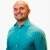# SAT: how to use the ratio to determine the correct data sampleThis lesson will look at an example for the SAT. The given information will assist in learning how to use the ratio to determine the correct data sample. This lesson will give a step by step... This lesson will look at an example for the SAT. The given information will assist in learning how to use the ratio to determine the correct data sample. This lesson will give a step by step explanation to better explain the concept.
More... Collapse
6 Views